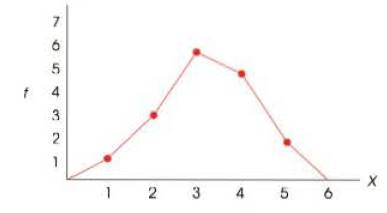Chapter 2, Problem 16PEssentials of Statistics for the B...

8th Edition
Frederick J Gravetter + 1 other
ISBN: 9781133956570

Solutions

Chapter
SectionEssentials of Statistics for the B...

8th Edition
Frederick J Gravetter + 1 other
ISBN: 9781133956570
Textbook Problem

Find each or the followin.tt values for the distribution shown in the following polygon. a. n b. ΣX c. ΣX2a.

To determine

To Find: The n value for the frequency distribution.

Explanation

Given info:

The frequency polygon is given in the question.

Justification:

The value of n is the sum of frequencies showing in the given frequency polygon. Just add all the frequencies showing by the red dots in the given frequency polygon:

f

b.

To determine

To Find: The X value for the frequency distribution.

c.

To determine

To Find: The X2 value for the frequency distribution.

Still sussing out bartleby?

Check out a sample textbook solution.

See a sample solution

The Solution to Your Study Problems

Bartleby provides explanations to thousands of textbook problems written by our experts, many with advanced degrees!

Get Started

In Exercises 75-98, perform the indicated operations and/or simplify each expression. 92. (3x + 2)(2 3x)

Applied Calculus for the Managerial, Life, and Social Sciences: A Brief Approach

1. True or false: (a) (b) (c) (d)

Mathematical Applications for the Management, Life, and Social Sciences

Rewrite 10x2xdx using a trigonometric substitution. a) cos10sind b) 110sincos2d c) 10cos2sind d) 10sincosd

Study Guide for Stewart's Single Variable Calculus: Early Transcendentals, 8th